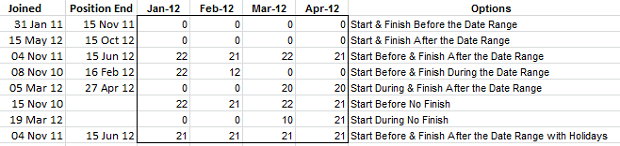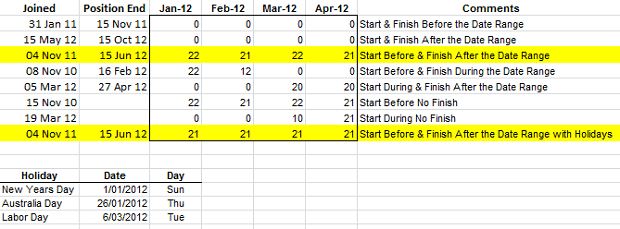# Formula Forensic 020. Bhavik’s Monthly Workingdays Formula

I recently received an email from Bhavik who was excited to share a neat little formula for determining the number of days worked during a month.

This formula has a wide range of uses in accounting, payroll, staff scheduling, accommodation planning & reporting and general scheduling activities.

Bhavik’s formula,

` =MAX(0,NETWORKDAYS(MAX(\$A2,C\$1),MIN(\$B2,EOMONTH(C\$1,0))))`

cleverly uses the Networkdays( ) function in conjunction with Min( ), Max( ) and Eomonth( ) functions to calculate the number of working days available during the month.

So today at Formula Forensics we will look at Bhavik’s Formula and see what makes it tick.

## Bhavik’s Formula

Bhavik’s Formula is based around the Excel Networkdays( ) function, which is wrapped inside a Max ( ) function and uses a couple of other Min( ), Max( ) and Eomonth( ) functions as part of the Networkdays( ) parameters.

`=MAX(0,NETWORKDAYS(MAX(\$A2,C\$1),MIN(\$B2,EOMONTH(C\$1,0))))`

Lets jump in and see what makes Bhavik’s Formula tick.

```=MAX(0,NETWORKDAYS(MAX(\$A2,C\$1),MIN(\$B2,EOMONTH(C\$1,0))))
```

### Caution

Instead of using the cell Ranges in describing the functionality of the file, I am going to use the following words

Join Date:            The Date that the employee Joined the company, Column A

Finish Date:        The Date that the employee’s position ended with the company, Column B

Month:                    The current Month’s Date. The month’s are described as dates using the first of the month as a Reference date. Eg: April 2012 = 1/4/2012

So to rewrite Bhavik’s Formula we using the above names (These aren’t Named Formulas)

`=MAX(0, NETWORKDAYS(MAX(Join Date, Month), MIN(Finish Date , EOMONTH(Month, 0))))`

Bhavik’s Formula is based around the Excel Networkdays( ) function.

The syntax for the Networkdays( ) function is shown below:We can see that the Networkdays( ) function has 2 requirements , being the Start Date and End Date

In Bhavik’s Formula:

`NETWORKDAYS(MAX(Join Date, Month), MIN(Finish Date , EOMONTH(Month, 0)))`

Start_Date:         MAX(Join Date, Month)

End_Date:           MIN(Finish Date , EOMONTH(Month, 0))

We can see that Bhavik’s Formula hasn’t included the optional Holidays functionality of the Networkdays( ) function. This will be discussed later.

Networkdays returns the number of work days between the Start Date and the End Date.

### Start Date

In Bhavik’s Formula, the Start Date is defined as MAX(Join Date, Month)

This will select the later date or highest of the Join Date and the Current Month. Hence if the Join Date is before the current month, the Current Month will be used as the start date.

If the Join Date is during the Current Month, the Join Date will be used as it will be higher than the Current month.

If the Join Date is after the Current Month, Networkdays will return a negative. This is dealt with by the leading Max(0, ) function which will take a 0 value if Networkdays  returns a negative number as any negative number is less than zero.

`=MAX(0, NETWORKDAYS(MAX(Join Date,Month), MIN(Fisnish Date , EOMONTH(Month, 0)))) `

### End Date

`=MAX(0, NETWORKDAYS(MAX(Join Date,Month), MIN(Finish Date , EOMONTH(Month, 0))))`

In Bhavik’s Formula, the End Date is defined as MIN(Finish Date , EOMONTH(Month, 0))

This will select the minimum date of the Finish Date or End of the Current Month, which is determined by the function, EOMONTH(Month, 0).

If the Finish Date is before the current month, the Finish Date will be used as the End Date. This may cause Networkdays to return a –‘ve which is corrected by the Max(0 as descried above:

`=MAX(0, NETWORKDAYS(MAX(Join Date, Month), MIN(Finish Date , EOMONTH(Month, 0))))`

If the Finish Date is during the current month, the Finish Date will be used as the End Date.

If the Finish Date is after the current month, the End of Month of the Current Month will be used as the End Date.

## Application

Now we understand how the formula works we can have a look at it in use :

In cell C2 put:

`=MAX(0,NETWORKDAYS(MAX(\$A2,C\$1),MIN(\$B2,EOMONTH(C\$1,0))))`

Copy across and downThere is an example of every date combination described above and listed in the Options Column.

Now that you understand the logic, You can work through these cells, one by one, and examine why each month’s formula works.

## Holidays

In the syntax of the Networkdays( ) function, you will see there is an optional Holidays parameter.

This can be as simple as a range of cells or an array of dates defining the holidays

In the worked example the range B13:B15 contains 3 dates reflecting 3 Public Holidays in Australia.

When the dates are added to the formula

`=MAX(0,NETWORKDAYS(MAX(\$A9,C\$1),MIN(\$B9,EOMONTH(C\$1,0)),\$B\$13:\$B\$15))`

We can see that the workdays in January and March are reduced by 1 day each, noting that New Years Day is on a Sunday and hence not included as a Holiday.I should note that in Australia the New Years Day holiday is actually taken on the following Monday (2 Jan 2012), but this was excluded for this example as a demonstration only to show that because it is on a Sunday it is not included.

## New Functions !

In Excel 2010, Microsoft introduced the Networkdays.intl( ) function.

This is a new version of the Networkdays Functionality but has the added benefit of being able to define the Weekends.

The Excel Networkdays.intl( ) function uses the following syntax:The main benefit of using  the Excel Networkdays.intl( ) function is that you can define your own weekends, rather than rely on the standard Saturday/Sunday option that Networkdays( ) provides.

For example:

`=MAX(0,NETWORKDAYS.INTL(MAX(\$A9,C\$1),MIN(\$B9,EOMONTH(C\$1,0)), 6, \$B\$13:\$B\$15))`

The weekend parameter is set to 6 and so excel will use Thursday and Friday as the weekend

`=MAX(0,NETWORKDAYS.INTL(MAX(\$A9,C\$1),MIN(\$B9,EOMONTH(C\$1,0)), "1010100", \$B\$13:\$B\$15))`

The weekend parameter is set to a text string of “1010100” and so excel will use Monday, Wednesday and Friday as the weekend days

`=MAX(0,NETWORKDAYS.INTL(MAX(\$A9,C\$1),MIN(\$B9,EOMONTH(C\$1,0)), Z1, \$B\$13:\$B\$15))`

The weekend parameter is set to Z1 and so Excel will retrieve the value from cell Z1 to define the weekend. Cell Z1 must contain a valid number from 1 to 17 as described above or a 7 character Text string like ‘1010100.

I strongly recommend that users who have switched to Excel 2010 start using the new formulas, as they add a raft of new features to your Excel arsenal.

### What else is new in Excel 2010?

What else is new in Excel 2010? Have a look here.

## Formula Forensics “The Series”

This is the 20th post in the Formula Forensics series.

Formula Forensic Series

## Formula Forensics Needs Your Help !

I need more ideas for future Formula Forensics posts and so I continue to need your help.

If you have a neat formula that you would like to share and explain, try putting pen to paper and draft up a Post like above or;

If you have a formula that you would like explained but don’t want to write a post as Bhavik’s has done here, send it to Hui or Chandoo.Hello Awesome...

My name is Chandoo. Thanks for dropping by. My mission is to make you awesome in Excel & your work. I live in Wellington, New Zealand. When I am not F9ing my formulas, I cycle, cook or play lego with my kids. Know more about me.

Thank you and see you around.

### Related articles:

 Written by Hui... Tags: advanced excel, date and time, eomonth, Learn Excel, max(), Microsoft Excel Formulas, MIN(), networkdays(), Networkdays.intl() Home: Chandoo.org Main Page ? Doubt: Ask an Excel Question

### 7 Responses to “Formula Forensic 020. Bhavik’s Monthly Workingdays Formula”

1.ahmed says:

This is a great formula, but it considered weekdays to be Saturday and Sunday, by default.

How can it be changed for weekdays to be Friday and Saturday as in my country?

•Hui... says:

Ahmed
Did you read the section on Networkdays.Intl ?
It does exactly what you want.

2.Planilhas Excel says:

I'm glad they added this function to Excel 2010, as it is very easy to make mistakes when making such a calculation

3.Cameron says:

I had someone recently ask for number of working days between two dates by weekday.

Here's what I came up with; I would be interested to see if anyone has a different solution:

[Uses two system tables, Semesters and Holidays]

[Semesters has 10 columns:

Semester - The name of the date range (obviously a school semester in my example, but not necessarily so.)
start - the first date in the date range
end - the last date in the date range
Sunday - shows the number of Sundays in the date range, excluding Sunday holidays
Monday - you get the idea.
Tuesday
Wednesday
Thursday
Friday
Saturday]

[Holidays has 1 column:

List - just a list of dates representing holidays]

[Semesters formulas as follows:

Sunday: =SUMPRODUCT(--(TEXT([@start]-1+ROW(OFFSET(\$B\$1,0,0,[@end]-[@start],1)),"dddd")="Sunday"))-SUMPRODUCT((Holidays[List]>=[@start])*(Holidays[List]<=[@end])*(TEXT(Holidays[List],"dddd")="Sunday"))
Monday: =SUMPRODUCT(--(TEXT([@start]-1+ROW(OFFSET(\$B\$1,0,0,[@end]-[@start],1)),"dddd")="Monday"))-SUMPRODUCT((Holidays[List]>=[@start])*(Holidays[List]<=[@end])*(TEXT(Holidays[List],"dddd")="Monday"))
Tuesday: Ok you get the idea again.
...]

I get the feeling that there's a cleaner, yet more abstract method to get these results?

Here's the file: http://www.vertexvortex.com/r/excel/Workdays-Weekdays.xlsx

4.Bob says:

I am trying to use the following (copied it from this post). =MAX(0,NETWORKDAYS.INTL(MAX(\$B21,D\$20),MIN(\$C21,EOMONTH(D\$20,0)),1,\$C\$32:\$C\$34))
What need to do is has the data calculate to a status date vs. going on if there is no Finish date.
I have been working on it fro 2 days now and need some help.  Can anyone help me please.
Thank you in advance for any and all help...

5.MamaG says:

I am attempting to make a calendar that will allow me to enter a date and then it will calculate for me the date that is 60 days before that date, 45 days before the date entered, 60 days after the date entered, and 120 days after the date entered. And it needs to exclude weekends and federal holidays for some of these time periods. Any ideas?

•Hui... says:

@MamaG
Try using the Workdays.Intl() function
=WORKDAY.INTL(A1,-60,1,Holidays)
Where A1 is the Date
-60 is 60 days before
1 is Sat/Sun weekends
Holidays is a Named Range with a list of Valid Holidays

 « Excel Links – Live from Sydney Edition Displaying Text Values in Pivot Tables without VBA »

### Get FREE Excel & Power-BI Newsletter

One email per week with Excel and Power BI goodness. Join 100,000+ others and get it free.